# Minimization of the Number of Iterations in K-Medoids Clustering with Purity Algorithm

Minimization of the Number of Iterations in K-Medoids Clustering with Purity Algorithm
Department of Informatics, Universitas Malikussaleh, Aceh 24353, Indonesia

Department of Informatics, Universitas Islam Kebangsaan Indonesia, Aceh 24352, Indonesia

Corresponding Author Email:
rozzi@unimal.ac.id
Page:
193-199
|
DOI:
https://doi.org/10.18280/ria.350302
11 April 2021
|
Accepted:
20 June 2021
|
Published:
30 June 2021
| Citation

OPEN ACCESS

Abstract:

With k-medoids algorithm, it often takes many iterations to cluster a large dataset, that is, the k-medoids algorithm cannot achieve the optimal performance. Based on cluster validity, this paper tries to optimize the clustering performance of k-medoids algorithm, using the purity algorithm. Specifically, the medoids value was determined by the purity value, and cluster validity was measured with the Davies Bouldin Index (DBI) on the Iris Dataset and the Death/Birth Rate Dataset. The results show that the cluster validity of the proposed purity k-medoids algorithm was better than the conventional k-medoids algorithm. The conventional k-medoids converged in an average of 8.7 iterations on the Death Birth Rate Dataset and 13.2 on the Iris Dataset. By contrast, the purity k-medoids algorithm only needed 2 iterations on either dataset. Therefore, the purity k-medoids algorithm can effectively minimize the number of iterations in the clustering of large datasets.

Keywords:

clustering, iteration, K-medoids, purity, Davies-Bouldin Index

1. Introduction

Machine learning is the study of computer algorithms and statistical models that execute tasks without explicit instructions . K-medoids, a.k.a. partitioning around medoids (PAM), is one of the machine learning algorithms relying on unsupervised learning. As a variant of the k-means algorithm, k-medoids provides a non-hierarchical clustering tool. In essence, data clustering is to divide data into several clusters (groups), trying to achieve the maximum intra-cluster similarity and minimum inter-cluster similarity [2, 3]. During the clustering tasks, k-medoids algorithm faces a serious problem: the number of iterations is unpredictable and instable . If a large volume of data needs to be processed, the algorithm could hardly achieve the optimal performance.

Some scholars have studied the performance of k-medoids in data clustering. Arora and Varshney  clustered the big data separately with k-means and k-medoids, and found that k-medoids was superior in terms of execution time, insensitivity to outliers, and noise suppression. Gulo et al.  introduced k-medoids to the clustering of uncertain data, and demonstrated the high accuracy and efficiency of the algorithm. Tiwari et al.  proposed the k-medoids with multi-armed bandits (banditPAM), learned that banditPAM could produce high-quality medoids on huge data, and applied k-medoids to improve the quality of online learning for students around the world. Schubert and Rousseeuw  analyzed fast and eager k-medoids clustering, observed that clustering large applications (CLARA) achieved better quality than random medoids, and proved that FasterPAM worked faster and yielded better results than conventional k-medoids. Velmurugan and Santhanam  proved that k-means algorithm consumed a longer mean computing time than k-medoids on data obeying normal or uniform distribution. In addition, a few researchers tried to optimize k-medoids with purity algorithm, which determines the medoids values for data clustering.

This paper mainly attempts to reduce the number of iterations required by conventional k-medoids with purity algorithm. One of the key defects of k-medoids is the random selection of medoids, which makes the number of iterations unpredictable. If the clustering takes many iterations, the execution time of the algorithm will be excessively long. To minimize the number of iterations, purity algorithm was introduced to determine the cluster medoids based on the purity values. Then, the authors conducted a test with three different k values to analyze how purity affects the number of iterations in different clusters. In addition, the results of our purity k-medoids were compared with those of conventional k-medoids. The clustering performance of the proposed algorithm was evaluated with the Davies-Bouldin Index (DBI), which measures the clustering effect with cohesion and separation. The DBI value is negatively correlated with the clustering effect . The datasets adopted for this study include the Iris Dataset from the UCI Machine Learning Repository and the Death Birth Rate Dataset from the John Burkardt Repository.

2. K-Medoids Clustering

K-Medoids is a non-hierarchical clustering algorithm K-medoids is a non-hierarchical clustering algorithm derived from the k-means algorithm. Through partition clustering, the algorithm groups x objects into k clusters. At the center of each cluster, the objects that are robust against outliers are called medoids. The clusters are formed by computing the distance between medoids and non-medoids objects .

As shown in Figure 1, k-medoids algorithm works in the following steps :

Step 1. Determine the k value (number of clusters);

Step 2. Initialize k random medoids in n data;

Step 3. Compute the Euclidean distance d(xi, µj) of each object xi to each medoid µj:

$d(x i, \mu j)=\sum_{i=1}^{n}(x i-\mu j)^{2}$      (1)

where, µj is the value of the medoid (center) the j-th cluster.

## 1.png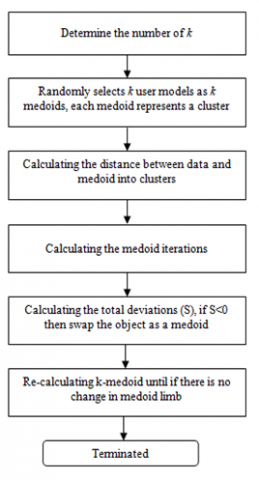Figure 1. Framework of K-medoids clustering

Step 4. Assign the object to the cluster of the closest medoid;

Step 5. Repeat the above steps, and calculate the total deviation (S):

$\mathrm{S}=\mathrm{b}-\mathrm{a}$     (2)

where, a is the sum of the closest distances between the object and the initial medoid; b is the sum of the closest distances between the object to the new medoid.

If S <0, then swap the object with the other data to form a new k as a medoid.

Step 6. Repeat Steps 3-5, and terminate the algorithm if the medoids change no more.

2.1 Purity algorithm

Purity algorithm aims to find the purity value of each cluster, i.e., the most suitable member in the cluster. The purity value can be calculated by :

$\operatorname{Purity}(y)=\frac{1}{N_{y}} \max \left(n_{x y}\right)$      (3)

where, purity (y) is the purity value of the y-th variable; Ny is the amount of data belonging to the y-th cluster; y is the index of the cluster.

2.2 DBI

This paper uses the DBI to analyze the clustering results of machine learning algorithms. This index measures clustering effect with cohesion and separation. The former is defined as the amount of data close to the centroid in each cluster, and the latter as the proximity between centroids in the cluster. The DBI can be computed in the following steps :

Step 1. Compute the sum of squares within cluster (SSW), i.e., cohesion, in a cluster by :

$S S W i=\frac{1}{m i} \sum_{j=i}^{m i} d(x j, c i)$     (4)

Step 2. Compute the sum of squares between clusters (SSB), i.e., separation, between clusters by :

$S S B i, j=d(c i, c j)$      (5)

Step 3. Compute the ratio that compares the i-th cluster with the j-th cluster :

$R i j=\frac{S S W i+S S W j}{S S B i j}$      (6)

Step 4. Compute the DBI based on the ratio by :

$D B I=\frac{1}{k} \sum_{i=1}^{k} \max _{i \neq j}\left(R_{i, j}\right)$     (7)

The smaller the DBI value, the better the clustering effect .

3. Purity K-Medoids

To improve the clustering quality, it is necessary to determine the medoids in k-medoids algorithm. This paper decides to determine them in the following procedure: First, the purity value is calculated for the entire dataset. Then, the highest and lowest purity values are taken as the medoids for k-medoids algorithm. Figure 2 shows the architecture of the k-medoids algorithm with the medoids selected by our approach.

The workflow of our research can be summarized as follows (Figure 3):

Step 1. Import the Iris Dataset and Death Birth Rate Dataset.

Step 2. Initialize the k value.

Step 3. Compute the purity value of each data by formula (3). If k value is even, then use the minimum purity value for the first iteration; if k value is odd, then use the maximum purity value for the first iteration.

Step 4. Compute the distance of each data to each medoid, and classify the data by formula (1).

Step 5. Compute the deviation S.

Step 6. Compute the DBI value, and analyze the results by formulas (4)-(7).

Step 7. Compare purity k-medoids with conventional k-medoids by analyzing the number of iterations and the DBI value (a metric of cluster validity).

## 2.png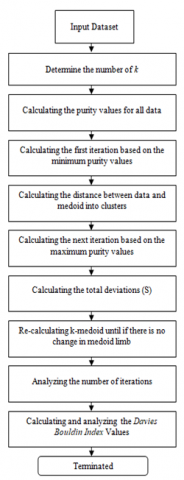Figure 2. Purity K-medoids

## 3.png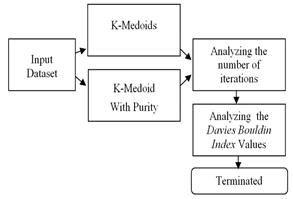Figure 3. Research framework

4. Results and Discussion

This research uses two different datasets, namely, the Iris Dataset from UCI Machine Learning Repository and the Death Birth Rate Dataset from John Burkardt Repository. The datasets are described in Table 1.

Table 1. The description of the dataset

 Dataset Number of Attributes Number of Instances DeathBirth Rates dataset 2 70 Iris dataset 4 150

4.1 Purity value on the two datasets

The purity value was computed with the above-mentioned purity value formula. The purity value of each data in the Death Birth Rate Dataset can be calculated by:

\begin{aligned} \text { 1. Purity }(d 1) &=\frac{1}{(51)}(36,4) \\ &=0.713725 \\ \text { 2. Purity }(d 2) &=\frac{1}{(45,3)}(37,3) \\ &=0.8234 \\ \text { 3. Purity }(d 3) &=\frac{1}{(57,4)}(42,1) \\ &=0.733449 \end{aligned}

Tables 2 and 3 present the purity values on the Death Birth Rate Dataset and the Iris Dataset, respectively.

Table 2. Purity values on the Death Birth Rate dataset

 Data No- Attribute 1 (x1) Attribute 2 (x2) Purity Value 1 36.4 14.6 0.713725 2 37.3 8 0.8234 3 42.1 15.3 0.733449 4 55.8 25.6 0.685504 ... ... ... ... 19 46.3 6.4 0.878558 ... ... ... ... 50 17.6 19.8 0.529412 ... ... ... ... 70 25.5 8.8 0.74344

Table 3. Purity values on the Iris dataset

 Data No- (x1) (x2) (x3) (x4) Purity Value 1 5.1 3.5 1.4 0.2 0.5 2 4.9 3 1.4 0.2 0.515789 3 4.7 3.2 1.3 0.2 0.5 4 4.6 3.1 1.5 0.2 0.489362 ... ... ... ... 42 4.5 2.3 1.3 0.3 0.535714 ... ... ... ... 101 6.3 3.3 6 2.5 0.348066 ... ... ... ... 150 5.9 3 5.1 1.8 0.373418

On the Death Birth Rate Dataset, the minimum purity value was 0.529412 on the 50th data, while the maximum purity value was 0.878558 on the 19th data. On the Iris Dataset, the minimum purity value was 0.348066 on the 101st data, while the maximum purity value was 0.535714 on the 42nd data.

In this research, the data with the minimum purity value is taken as the initial medoid, and that with the maximum purity value as the new medoid. Then, the clustering was initiated with different initial k values: 2, 3, and 4. After that, the clustering results were analyzed by comparing the DBI values

4.2 Clustering with K-Medoids

Ten tests were carried out to cluster the datasets with purity k-medoids, using the initial k value of 2. The Euclidean distance of each data to the initial medoid in the first iteration was computed.

\begin{aligned} \text { 1. } \mathrm{d} 1, \mathrm{~d} 1 &=\sqrt{(36,4-17,6)^{2}+(14,6-19,8)^{2}} \\ &=19,5059 \\ \text { 2. } \mathrm{d} 2, \mathrm{~d} 1 &=\sqrt{(36,4-17,5)^{2}+(14,6-13,7)^{2}} \\ &=18,92142 \\ \text { 3. } \mathrm{d} 1, \mathrm{~d} 2 &=\sqrt{(37,3-17,5)^{2}+(8-19,8)^{2}} \\ &=22,96367 \\ \text { 4. } \mathrm{d} 2, \mathrm{~d} 2 &=\sqrt{(36,4-17,5)^{2}+(14,6-13,7)^{2}} \\ &=20,60413 \\ \text { 5. } \mathrm{d} 1, \mathrm{~d} 3 &=\sqrt{(42,1-17,6)^{2}+(15,3-19,8)^{2}} \\ &=24,90984 \\ \text { 6. } \mathrm{d} 2, \mathrm{~d} 3 &=\sqrt{(36,4-17,5)^{2}+(14,6-13,7)^{2}} \\ &=24,65198 \end{aligned}

The following are the computed results (Table 4):

Table 4. Euclidean Distance of each data

 Data No- Distance Value dx,dx dx,dy 1 19,5059 18,92142 2 22,96367 20,60413 3 24,90984 24,65198 4 38,63781 40,10611 5 40,73254 43,20093 6 24,52835 24,39057 7 28,52122 29,03377 8 25,97884 24,4663 … … … 70 13,54289 9,381365

Next, the distance of each data to a new medoid was computed, and the total deviation (S) was derived by formula (2):

\begin{aligned} S &=b-a \\ &=564,1132-947,1384 \\ &=-383,0252 \end{aligned}

On this basis, the distance in that iteration was re-calculated until the medoids change no more. The clustering results of the conventional k-medoids and the purity k-medoids on Death Birth Rate Dataset with k=2 are contrasted in Table 5.

As shown in Table 5 and Figure 4, the conventional k-medoids required different number of iterations from test to test on Death Birth Rate Dataset. The highest number of iterations was 7 during the 7th and 8th tests, while the lowest was 3 during the 5th test. By contrast, the purity k-medoids algorithm took 2 iterations to complete the clustering. On average, the conventional k-medoids needed 5 iterations during the 10 tests, while the purity k-medoids needed 2 iterations.

Table 5. Clustering results of the conventional k-medoids and the Purity K-medoids on Death Birth Rate Dataset with k=2

 Test No Number of Iterations Conventional K-Medoids Purity K-Medoids 1 4 2 2 6 2 3 4 2 4 6 2 5 3 2 6 4 2 7 7 2 8 7 2 9 5 2 10 4 2 Average 5 2

## 4.png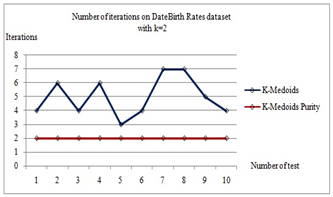Figure 4. Number of iterations of k-medoids clustering with k= 2 on Death Birth Rate Dataset

Next, the authors compared the clustering results of conventional k-medoids and purity k-medoids on the Iris Dataset with k=2.

Table 6. Clustering results of conventional k-medoids and Purity K-medoids on Iris Dataset with k=2

 Test No Number of Iterations Conventional K-Medoids PurityK-Medoids 1 7 2 2 10 2 3 4 2 4 6 2 5 3 2 6 10 2 7 7 2 8 7 2 9 5 2 10 10 2 Average 6.9 2

As shown in Table 6 and Figure 5, the conventional k-medoids required different number of iterations from test to test on Iris Dataset. The highest number of iterations was 10 during the 2nd, 6th, and 10th tests, while the lowest was 3 during the 3rd test. By contrast, the purity k-medoids algorithm took 2 iterations to complete the clustering. On average, the conventional k-medoids needed 6.9 iterations during the 10 tests, while the purity k-medoids needed 2 iterations.

## 5.png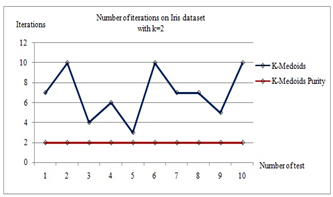Figure 5. Number of iterations of k-medoids clustering with k= 2 on Iris Dataset

After that, conventional k-medoids algorithm and purity k-medoids algorithm were applied separately to cluster Death Birth Rate Dataset with k=3.

Table 7. Clustering results of the conventional k-medoids and the purity k-medoids on Death Birth Rate Dataset with k=3

 Test No Number of Iterations Conventional K-Medoids Purity K-Medoids 1 7 2 2 7 2 3 5 2 4 9 2 5 7 2 6 5 2 7 5 2 8 7 2 9 5 2 10 9 2 Average 6.6 2

## 6.pngFigure 6. Number of iterations of k-medoids clustering with k= 3 on Death Birth Rate Dataset

As shown in Table 7 and Figure 6, the conventional k-medoids required different number of iterations from test to test on Death Birth Rate Dataset. The highest number of iterations was 9 during the 4th, and 10th tests, while the lowest was 5 during the 3rd, 6th, 7th, and 9th test. By contrast, the purity k-medoids algorithm took 2 iterations to complete the clustering. On average, the conventional k-medoids needed 6.6 iterations during the 10 tests, while the purity k-medoids needed 2 iterations.

Next, the authors compared the clustering results of conventional k-medoids and purity k-medoids on the Iris Dataset with k=3.

Table 8. Clustering results of conventional k-medoids and purity k-medoids on Iris Dataset with k=3

 Test No Number of Iterations Conventional K-Medoids Purity K-Medoids 1 13 2 2 17 2 3 10 2 4 13 2 5 16 2 6 9 2 7 17 2 8 10 2 9 16 2 10 13 2 Average 13.4 2

As shown in Table 8 and Figure 7, the conventional k-medoids required different number of iterations from test to test on Iris Dataset. The highest number of iterations was 17 during the 2nd, and 7th tests, while the lowest was 9 during the 6th test. By contrast, the purity k-medoids algorithm took 2 iterations to complete the clustering. On average, the conventional k-medoids needed 13.4 iterations during the 10 tests, while the purity k-medoids needed 2 iterations.

## 7.png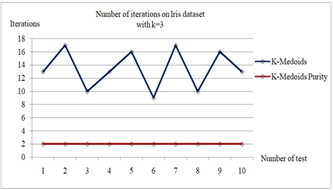Figure 7. Number of iterations of k-medoids clustering with k= 3 on Iris Dataset

Table 9. Clustering results of the conventional k-medoids and the purity k-medoids on Death Birth Rate Dataset with k=4

 Test No Number of Iterations Conventional K-Medoids Purity K-Medoids 1 15 2 2 18 2 3 14 2 4 13 2 5 17 2 6 15 2 7 11 2 8 13 2 9 14 2 10 17 2 Average 14.7 2

Furthermore, conventional k-medoids algorithm and purity k-medoids algorithm were applied separately to cluster Death Birth Rate Dataset with k=4.

As shown in Table 9 and Figure 8, the conventional k-medoids required different number of iterations from test to test on Death Birth Rate Dataset. The highest number of iterations was 18 during the 2nd test, while the lowest was 11 during the 7th test. By contrast, the purity k-medoids algorithm took 2 iterations to complete the clustering. On average, the conventional k-medoids needed 14.7 iterations during the 10 tests, while the purity k-medoids needed 2 iterations.

## 8.png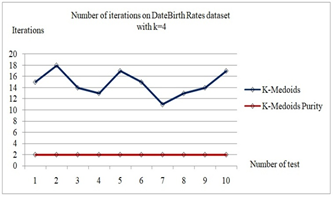Figure 8. Number of iterations of k-medoids clustering with k= 4 on Death Birth Rate Dataset

Next, the authors compared the clustering results of conventional k-medoids and purity k-medoids on the Iris Dataset with k=4.

Table 10. Clustering results of conventional k-medoids and purity k-medoids on Iris Dataset with k=4

 Test No Number of Iterations Conventional K-Medoids Purity K-Medoids 1 23 2 2 18 2 3 15 2 4 19 2 5 19 2 6 21 2 7 17 2 8 26 2 9 20 2 10 17 2 Average 19.5 2

## 9.png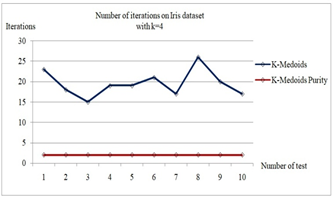Figure 9. Number of iterations of k-medoids clustering with k= 4 on Iris Dataset

As shown in Table 10 and Figure 9, the conventional k-medoids required different number of iterations from test to test on Iris Dataset. The highest number of iterations was 26 during the 8th test, while the lowest was 15 during the 3rd test. By contrast, the purity k-medoids algorithm took 2 iterations to complete the clustering. On average, the conventional k-medoids needed 19.5 iterations during the 10 tests, while the purity k-medoids needed 2 iterations.

4.3 Results of DBI

Table 11 show the validation results of the DBI values for the clustering by conventional k-medoids and purity k-medoids, respectively.

Table 11. Validation results for the DBI values

 No Dataset Number of K Davies-Bouldin Index (DBI) Value Conventional K-Medoids Purity K-Medoids 1 DeathBirth Rates Dataset 2 0.8821 0.6719 3 1.5128 0.7847 4 1.9275 0.9015 2 Iris Dataset 2 1.1079 0.6612 3 1.8771 0.8103 4 2.4799 0.9571

## 10.png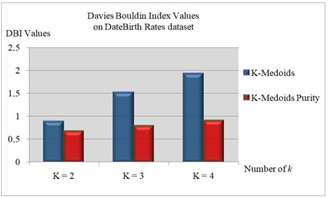Figure 10. DBI values on Death Birth Rate Dataset

## 11.png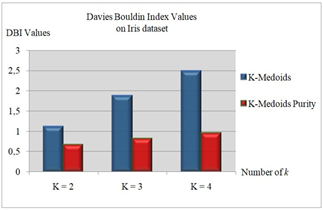Figure 11. DBI values on Iris Dataset

As shown in Table 11, the DBI values obtained from the two datasets were tested by conventional and purity k-medoids algorithms for different k values: 2, 3, and 4. The DBI value on Death Birth Rate Dataset using conventional k-medoids were sequentially 0.8821, 1.5128, and 1.9275. Meanwhile, the DBI value on Death Birth Rate Dataset using purity k-medoids were sequentially 0.6719, 0.7847, and 0.9015. More details are provided in Figure 10.

On Iris Dataset, the DBI values obtained by conventional k-medoids were 1.1079, 1.8771, and 2.4799 sequentially. Those obtained by purity k-medoids were 0.6612, 0.8103, and 0.9571 sequentially. More details are provided in Figure 11.

5. Conclusions

This paper uses the purity algorithm to determine the medoids. In this way, the number of iterations in k-medoids clustering was minimized. Our results show that the conventional k-medoids algorithm took an average of 8.7 iterations to cluster Death Birth Rate Dataset, while that of the proposed purity k-medoids was only 2 iterations. On Iris Dataset, the average number of iterations of conventional k-medoids was 13.2, while that of purity k-medoids was 2. Hence, purity algorithm can greatly reduce the number of iterations of k-medoids clustering. In addition, DBI validation shows that purity k-medoids achieved better clustering effect on both datasets: the lowest DBI of purity k-medoids on Death Birth Rate Dataset was 0.6719, and that on Iris Dataset was 0.6612; the lowest DBI of conventional k-medoids on Death Birth Rate Dataset was 0.8821, and that on Iris Dataset was 1.1079.

References

 Hasdyna, N., Sianipar, B., Zamzami, E.M. (2020). Improving the performance of K-nearest neighbor algorithm by reducing the attributes of dataset using gain ratio. Journal of Physics: Conference Series, 1566(1): 012090.

 Martanto, Anwar, S., Rohmat, C.L., Basysyar, F.M., Wijaya, Y.A. (2021). Clustering of internet network usage using the K-Medoid method. IOP Conference Series: Materials Science and Engineering, 1088(1): 012036.

 Johnson, M.G., Pokorny, L., Dodsworth, S., Botigué, L.R., Cowan, R.S., Devault, A., Eiserhardt, W.L., Epitawalage, N., Forest, F., Kim, J.T., Leebens-Mack, J.H., Leitch, I.J., Maurin, O., Soltis, D.E., Soltis, P.S., Wong, G.K., Baker, W.J., Wickett, N.J. (2019). A universal probe set for targeted sequencing of 353 nuclear genes from any flowering plant designed using K-medoids clustering. Systematic Biology, 68(4): 594-606. https://doi.org/10.1093/sysbio/syy086

 Song, H., Lee, J.G., Han, W.S. (2017). PAMAE: Parallel k-medoids clustering with high accuracy and efficiency. In Proceedings of the 23rd ACM SIGKDD International Conference on Knowledge Discovery and Data Mining, pp. 1087-1096.

 Arora, P., Varshney, S. (2016). Analysis of K-means and K-medoids algorithm for big data. Procedia Computer Science, 78: 507-512. https://doi.org/10.1016/j.procs.2016.02.095

 Gullo, F., Ponti, G., Tagarelli, A. (2008). Clustering uncertain data via K-medoids. In: Greco S., Lukasiewicz T. (eds) Scalable Uncertainty Management. SUM 2008. Lecture Notes in Computer Science, vol 5291. Springer, Berlin, Heidelberg. https://doi.org/10.1007/978-3-540-87993-0_19

 Tiwari, M., Zhang, M.J., Mayclin, J., Thrun, S., Piech, C., Shomorony, I. (2020). Bandit-PAM: Almost linear time k-medoids clustering via multi-armed bandits. arXiv preprint arXiv:2006.06856.

 Schubert, E., Rousseeuw, P.J. (2020). Fast and eager k-medoids clustering: O(k) runtime improvement of the PAM, CLARA, and CLARANS algorithms. arXiv preprint arXiv:2008.05171.

 Velmurugan, T., Santhanam, T. (2010). Computational complexity between K-means and K-medoids clustering algorithms for normal and uniform distributions of data points. Journal of Computer Science, 6(3): 363-368. https://doi.org/10.3844/jcssp.2010.363.368

 Candoré, J.C., Bodnar, J.L., Detalle, V., Grossel, P. (2010). Non destructive testing in situ, of works of art by stimulated infra-red thermography. Journal of Physics: Conference Series, 214(1): 012068.

 Tempola, F., Assagaf, A.F. (2018). Clustering of potency of shrimp in Indonesia with k-means algorithm and validation of Davies-Bouldin index. In International Conference on Science and Technology (ICST 2018). Atlantis Press.

 Wu, L., Liang, J., Li, B. (2019). Large-scale agent data partitioning based on DensityRepel K_medoids. In Journal of Physics: Conference Series, 1284(1): 012046.

 Xiao, J., Lu, J., Li, X. (2017). Davies Bouldin Index based hierarchical initialization K-means. Intelligent Data Analysis, 21(6): 1327-1338. https://doi.org/10.3233/IDA-163129

 Praveena, S. (2018). Reduction of Davies Bouldin index using hybrid clustering algorithm and Naive Bayes Classifier. International Journal of Engineering, Science and Mathematics, 7(3): 327-329.

 Mughnyanti, M., Efendi, S., Zarlis, M. (2020). Analysis of determining centroid clustering x-means algorithm with Davies-Bouldin index evaluation. In IOP Conference Series: Materials Science and Engineering, 725(1): 012128.

 Ghufron, G., Surarso, B., Gernowo, R. (2020). The implementations of K-medoids clustering for higher education accreditation by evaluation of Davies Bouldin index clustering. Jurnal Ilmiah KURSOR, 10(3). https://doi.org/10.21107/kursor.v10i3.232

 Tsoi, K.K., Chan, N.B., Yiu, K.K., Poon, S.K., Lin, B., Ho, K. (2020). Machine learning clustering for blood pressure variability applied to Systolic Blood Pressure Intervention Trial (SPRINT) and the Hong Kong Community Cohort. Hypertension, 76(2): 569-576. https://doi.org/10.1161/HYPERTENSIONAHA.119.14213

 Gustriansyah, R., Suhandi, N., Antony, F. (2020). Clustering optimization in RFM analysis based on k-means. Indonesian Journal of Electrical Engineering and Computer Science, 18(1): 470-477. http://doi.org/10.11591/ijeecs.v18.i1.pp470-477

 Azhir, E., Navimipour, N.J., Hosseinzadeh, M., Sharifi, A., Darwesh, A. (2021). An automatic clustering technique for query plan recommendation. Information Sciences, 545: 620-632. https://doi.org/10.1016/j.ins.2020.09.037